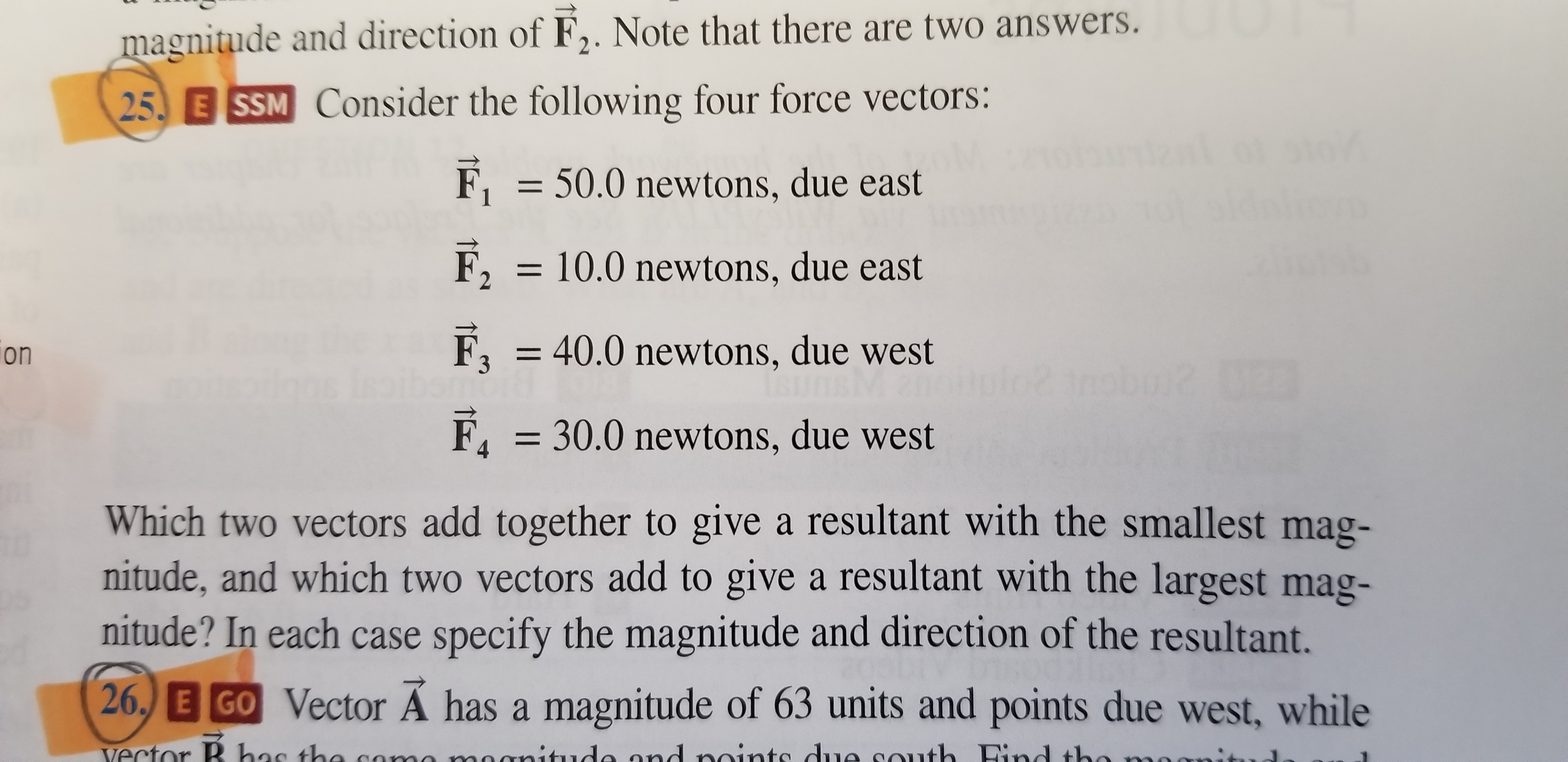# magnitude and direction of F2. Note that there are two answers. 25 E SSM Consider the following four force vectors: F150.0 newtons, due east F210.0 newtons, due east F3 40.0 newtons, due west F, 30.0 newtons, due west on Which two vectors add together to give a resultant with the smallest mag- nitude, and which two vectors add to give a resultant with the largest mag- nitude? In each case specify the magnitude and direction of the resultant. 26, E GO Vector Ä has a magnitude of 63 units and points due west, while

Question

Consider the following 4 force vectors:
50.0 Newton's, due east
10.0 Newton's, due east
40.0 Newton's, due west
30.0 Newton's, due west
Which 2 vectors add together to give a resultant with the smallest magnitude and which 2 vectors add to give a resultant with the largest magnitude ? In each case for specify the magnitude and direction of the resident resilient.help_outlineImage Transcriptionclosemagnitude and direction of F2. Note that there are two answers. 25 E SSM Consider the following four force vectors: F150.0 newtons, due east F210.0 newtons, due east F3 40.0 newtons, due west F, 30.0 newtons, due west on Which two vectors add together to give a resultant with the smallest mag- nitude, and which two vectors add to give a resultant with the largest mag- nitude? In each case specify the magnitude and direction of the resultant. 26, E GO Vector Ä has a magnitude of 63 units and points due west, while fullscreen

1 Rating

### Want to see this answer and more?

Experts are waiting 24/7 to provide step-by-step solutions in as fast as 30 minutes!*